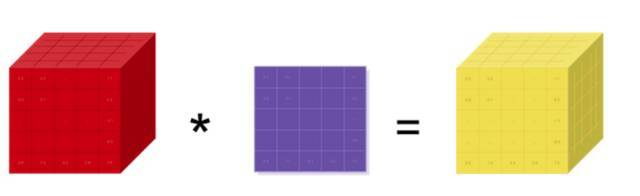Auto ByteScience AI# Yoshua Bengio实验室MILA开放面向初学者的PyTorch教程

MILA 实验室近日在 GitHub 上开启了一个初学者入门项目，旨在帮助 MILA 新生快速掌握机器学习相关的实践基础。目前该项目已经提供了一系列的 PyTorch 入门资料，并从张量、自动微分、图像识别、神经机器翻译和生成对抗网络等方面详细阐述。

PyTorch 是 Torch 在 Python 上的衍生，它本质上是 Numpy 的替代者，而且支持 GPU 加速深度神经网络的训练。如果读者熟悉 Numpy、Python 以及常见的深度学习概念（卷积层、循环层、SGD 等），那么我们可以容易上手 PyTorch。下面，我们将跟随 MILA 的教程共同探索 PyTorch 的基本概念与操作。

### Torch 张量库与基本操作``````torch.Tensor(5, 3)
---------------------------------------
2.4878e+04  4.5692e-41  2.4878e+04
4.5692e-41 -2.9205e+19  4.5691e-41
1.2277e-02  4.5692e-41 -4.0170e+19
4.5691e-41  1.2277e-02  4.5692e-41
0.0000e+00  0.0000e+00  0.0000e+00
[torch.FloatTensor of size 5x3]``````

``````torch.Tensor(5, 3).uniform_(-1, 1)
---------------------------------------------
-0.2767 -0.1082 -0.1339
-0.6477  0.3098  0.1642
-0.1125 -0.2104  0.8962
-0.6573  0.9669 -0.3806
0.8008 -0.3860  0.6816
[torch.FloatTensor of size 5x3]````````````>>> torch.FloatTensor([[1, 2, 3], [4, 5, 6]])
1  2  3
4  5  6
[torch.FloatTensor of size 2x3]
>>> print(x)
6.0
>>> x = 8
>>> print(x)
1  8  3
4  5  6
[torch.FloatTensor of size 2x3]``````

``````z = torch.LongTensor([[1, 3], [2, 9]])
print(z.type())
# Cast to numpy ndarray
print(z.numpy().dtype)
--------------------------------------------
torch.LongTensor
int64``````

``````x = torch.Tensor(5, 3).uniform_(-1, 1)
y = x * torch.randn(5, 3)
print(y)
---------------------------------------------
0.2200 -0.0368  0.4494
-0.2577 -0.0343  0.1587
-0.7503 -0.1729  0.0453
0.9296 -0.1067 -0.6402
-0.3276  0.0158 -0.0552
[torch.FloatTensor of size 5x3]``````

PyTorch 同样支持广播（Broadcasting）操作，一般它会隐式地把一个数组的异常维度调整到与另一个算子相匹配的维度以实现维度兼容。为了定义两个形状是否是可兼容的，PyTorch 会从最后开始往前逐个比较它们的维度大小。在这个过程中，如果两者的对应维度相同，或者其一（或者全是）等于 1，则继续进行比较，直到最前面的维度。若不满足这两个条件，程序就会报错。如下展示了 PyTorch 的广播操作：

``````print (x.size())
y = x + torch.randn(5, 1)
print(y)
---------------------------------------------
torch.Size([5, 3])
0.1919 -0.5006 -1.2410
-0.8080  0.1407 -0.6193
-1.6629 -0.1580 -0.3921
1.0395  0.7069 -0.1459
1.9027  1.4343  1.2299
[torch.FloatTensor of size 5x3]``````

``````y = torch.randn(5, 10, 15)
print(y.size())
print(y.view(-1, 15).size())  # Same as doing y.view(50, 15)
print(y.view(-1, 15).unsqueeze(1).size()) # Adds a dimension at index 1.
print(y.view(-1, 15).unsqueeze(1).squeeze().size())
# If input is of shape: (Ax1xBxCx1xD)(Ax1xBxCx1xD) then the out Tensor will be of shape: (AxBxCxD)(AxBxCxD)
print()
print(y.transpose(0, 1).size())
print(y.transpose(1, 2).size())
print(y.transpose(0, 1).transpose(1, 2).size())
print(y.permute(1, 2, 0).size())
-----------------------------------------------------
torch.Size([5, 10, 15])
torch.Size([50, 15])
torch.Size([50, 1, 15])
torch.Size([50, 15])
torch.Size([10, 5, 15])
torch.Size([5, 15, 10])
torch.Size([10, 15, 5])
torch.Size([10, 15, 5])``````

``````x = torch.cuda.HalfTensor(5, 3).uniform_(-1, 1)
y = torch.cuda.HalfTensor(3, 5).uniform_(-1, 1)
torch.matmul(x, y)
-----------------------------------------------------
0.2456  1.1543  0.5376  0.4358 -0.0369
0.8247 -0.4143 -0.7188  0.3953  0.2573
-0.1346  0.7329  0.5156  0.0864 -0.1349
-0.3555  0.3135  0.3921 -0.1428 -0.1368
-0.4385  0.5601  0.6533 -0.2793 -0.5220
[torch.cuda.HalfTensor of size 5x5 (GPU 0)]
# 以下转化CPU张量为GPU张量
x = torch.FloatTensor(5, 3).uniform_(-1, 1)
print(x)
x = x.cuda(device=0)
print(x)
x = x.cpu()
print(x)``````

### PyTorch 自动求导机制

PyTorch 文档地址：http://pytorch.org/docs/master/

TensorFlow、Caffe 和 CNTK 等大多数框架都是使用的静态计算图，开发者必须建立或定义一个神经网络，并重复使用相同的结构来执行模型训练。改变网络的模式就意味着我们必须从头开始设计并定义相关的模块。但是通过 PyTorch，我们使用了一种称为反向模式的自动微分技术（Reverse-mode auto-differentiation），它可以让开发者简单且低成本地改变神经网络的工作方式。按照文档所述，PyTorch 的灵感来自于自动微分的几篇研究论文，以及当前和过去的工作，如 autograd，Chainer 等。虽然这种技术并不是 PyTorch 独有的，但它是迄今为止最快的实现之一，因此它也为研究工作提供了最好的速度和灵活性。``````x = Variable(torch.Tensor(5, 3).uniform_(-1, 1), requires_grad=True)
y = Variable(torch.Tensor(5, 3).uniform_(-1, 1), requires_grad=True)
z = x ** 2 + 3 * y
# eq computes element-wise equality
----------------------------------------------------------------------
Variable containing:
1  1  1
1  1  1
1  1  1
1  1  1
1  1  1
[torch.ByteTensor of size 5x3]``````

``````y.grad
-------------------------------
Variable containing:
3  3  3
3  3  3
3  3  3
3  3  3
3  3  3
[torch.FloatTensor of size 5x3]``````

``````x = Variable(torch.Tensor(5, 3).uniform_(-1, 1), requires_grad=True)
y = Variable(torch.Tensor(5, 3).uniform_(-1, 1), requires_grad=True)
z = x ** 2 + 3 * y

• 线性层- nn.Linear、nn.Bilinear
• 卷积层 - nn.Conv1d、nn.Conv2d、nn.Conv3d、nn.ConvTranspose2d
• 非线性激活函数- nn.Sigmoid、nn.Tanh、nn.ReLU、nn.LeakyReLU
• 池化层 - nn.MaxPool1d、nn.AveragePool2d
• 循环网络 - nn.LSTM、nn.GRU
• 归一化 - nn.BatchNorm2d
• Dropout - nn.Dropout、nn.Dropout2d
• 嵌入 - nn.Embedding
• 损失函数 - nn.MSELoss、nn.CrossEntropyLoss、nn.NLLLoss

``````class Classifier(nn.Module):
"""Convnet Classifier"""
def __init__(self):
super(Classifier, self).__init__()
self.conv = nn.Sequential(
# Layer 1
nn.Dropout(p=0.5),
nn.ReLU(),
nn.MaxPool2d(kernel_size=(2, 2), stride=2),
# Layer 2
nn.Dropout(p=0.5),
nn.ReLU(),
nn.MaxPool2d(kernel_size=(2, 2), stride=2),
# Layer 3
nn.Dropout(p=0.5),
nn.ReLU(),
nn.MaxPool2d(kernel_size=(2, 2), stride=2),
# Layer 4
nn.Dropout(p=0.5),
nn.ReLU(),
nn.MaxPool2d(kernel_size=(2, 2), stride=2)
)
# Logistic Regression
self.clf = nn.Linear(128, 10)
def forward(self, x):
return self.clf(self.conv(x).squeeze())```````````` (deconv1): ConvTranspose2d(128, 128, kernel_size=(4, 4), stride=(1, 1), bias=False)
(bn1): BatchNorm2d(128, eps=1e-05, momentum=0.1, affine=True)
(deconv2): ConvTranspose2d(128, 64, kernel_size=(4, 4), stride=(2, 2), padding=(1, 1), bias=False)
(bn2): BatchNorm2d(64, eps=1e-05, momentum=0.1, affine=True)
(deconv3): ConvTranspose2d(64, 32, kernel_size=(4, 4), stride=(2, 2), padding=(1, 1), bias=False)
(bn3): BatchNorm2d(32, eps=1e-05, momentum=0.1, affine=True)
(deconv4): ConvTranspose2d(32, 1, kernel_size=(4, 4), stride=(2, 2), padding=(3, 3), bias=False)````````````(conv1): Conv2d(1, 32, kernel_size=(4, 4), stride=(2, 2), padding=(1, 1), bias=False)
(bn1): BatchNorm2d(32, eps=1e-05, momentum=0.1, affine=True)
(conv2): Conv2d(32, 64, kernel_size=(4, 4), stride=(2, 2), padding=(1, 1), bias=False)
(bn2): BatchNorm2d(64, eps=1e-05, momentum=0.1, affine=True)
(conv3): Conv2d(64, 128, kernel_size=(4, 4), stride=(2, 2), padding=(1, 1), bias=False)
(bn3): BatchNorm2d(128, eps=1e-05, momentum=0.1, affine=True)
(conv4): Conv2d(128, 1, kernel_size=(4, 4), stride=(2, 2), padding=(1, 1), bias=False)``````